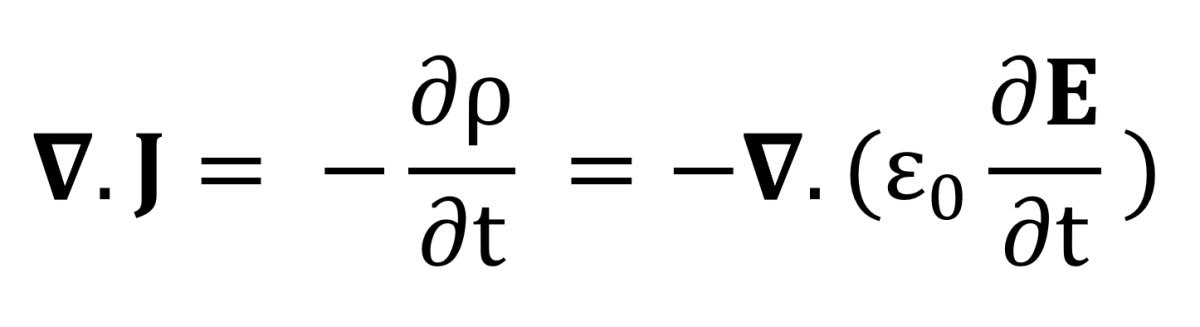# Maxwell's Equations and Displacement Current

Mohammad Yasir is a physics graduate from the University of Delhi and currently enrolled in the master's programme at IIT.

## Introduction

To say that James Clerk Maxwell revolutionized the world of Electrodynamics would be an understatement. The four equations named after him stand as a testament to his contribution to the field. While consolidated from the works of previous scientists, his correction to Ampere's law brought significant advancements into our understanding of the effects of dynamic charges, currents, and magnetic fields.

In fact, such is the power embedded within the four equations given above that together with the Lorentz Force Law, they suffice in explaining the entirety of electromagnetism on their own!

## Prerequisite

At the very least, the reader of this article must be comfortably acquainted with Ampere's law and the steady-state equations of electromagnetism. These include Gauss' law, Faraday's law, and the non-existence of magnetic monopoles, evidenced by the vanishing divergence of magnetic flux intensity, B.

Also, note that to differentiate vector quantities, I'll be using the bold typeface for representing them in this article.

## Electromagnetism Before Maxwell

When Maxwell began his work over a century ago, our entire knowledge of electromagnetism was limited to the abovementioned equations. Perhaps due to limited resources or some other reason, a very obvious problem escaped our attention until Maxwell pointed it out. Then again, for steady currents, the above equations served all purposes without the slightest fault. Either way, let us see how and where they fail.

## A "Fatal Flaw"

This situation arises when you try to find the divergence of the quantities on either side of the fourth equation. It is a well-known and mathematically sound fact that the divergence of the curl of a vector field is, without any exclusion, zero.

So far, so good. But wait! The right hand side of the above equation does not necessarily vanish. If we confine ourselves to the realm of steady currents, the divergence of current density J is zero. However, beyond the world of static charges and fields, the equation of continuity comes into the picture and we have ourselves, a paradox. Not only that, go through the following exercise and see if you can figure out another problem with Ampere's law.

## A Little Bit of Exercise

In the image attached above, I have drawn a capacitor connected to an emf source, and two Amperian loops, labelled 1 and 2.

1. A simple, circular loop around the wire.
2. A balloon-shaped structure that goes over one of the capacitor plates.
Scroll to Continue

Try applying Ampere's law to both of these loops and see what you find.

If you perform this exercise correctly, you will find that in the first case that the current enclosed is simply i, while in the second case, it comes out to be zero! This discrepancy is further evidence of the failure of Ampere's law in the case of non-steady currents since it was arrived at using Biot-Savart law.

## Fixing Ampere's LawThe equation of continuity can be used to relate current density with charge density, which is proportional to the divergence of time derivative of electric field via Gauss' law.

Now, you can intuitively see that if we add the extra term of ε0(∂E/∂t) to J in Ampere's law, we can easily remove the del operator and arrive at the following equation.

## Displacement Current

This is the modified form of Ampere's law, with the additional term of µ0ε0 (∂E/∂t). Note that in steady conditions where E is a constant in time, the equation reverts back to its original form.

### The value ε0 (∂E/∂t) was termed by Maxwell as Displacement Current.

But do not be misled since it is not a current in any way. The name arises from the fact that electric field can be related to the electric displacement vector via the relation D = ε0E + P, where P is the electric polarization vector.

Hence, we now have a more generalized form of the relation between the curl of magnetic flux intensity, current density, and electric field. In fact, the additional term we just figured out leads to a revolutionary realization.

A changing electric field induces a magnetic field, just like a changing magnetic field induces an electric field (Faraday's law).

## Conclusion

While what we've discussed is just a theoretical argument, albeit with a pleasant symmetry, it was proven experimentally too. Hertz's Experiments on Electromagnetic waves in 1888 showed that Maxwell was right all along, even though his reasons for using the quantity µ0ε0(E/∂t) were related to the now obsolete Ether model of the universe. The equation of continuity came into the picture as a happy coincidence but today, it is a far more compelling argument.

That completes our short discussion on Maxwell's equations and how he arrived at them. His correction to Ampere's law led to several revolutionary ideas and laid the foundation of modern electrodynamics.

This content is accurate and true to the best of the author’s knowledge and is not meant to substitute for formal and individualized advice from a qualified professional.